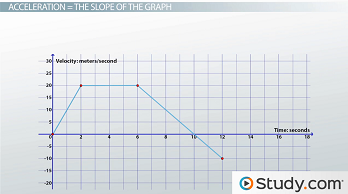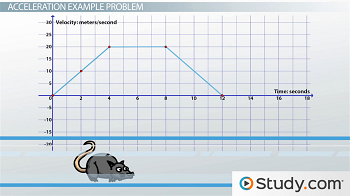# Determining Acceleration Using the Slope of a Velocity vs. Time Graph

Lesson Transcript
Instructor: Angela Hartsock

Angela has taught college microbiology and anatomy & physiology, has a doctoral degree in microbiology, and has worked as a post-doctoral research scholar for Pittsburgh’s National Energy Technology Laboratory.

In this lesson, we will learn how to use the slope of the line on a velocity vs. time graph to calculate the acceleration of an object in straight line motion.

## Kinematics with the X,Y Graph

The basic x, y graph has many interesting applications in physics and kinematics. In addition to succinctly describing the straight line motion of an object, you can use these graphs to calculate information like displacement, velocity, and acceleration. In this lesson, we'll continue our examination of the velocity vs. time graph and how it can be used to calculate the acceleration of an object in straight line motion.

An error occurred trying to load this video.

Try refreshing the page, or contact customer support.

Coming up next: Velocity vs. Time: Determining Displacement of an Object

### You're on a roll. Keep up the good work!

Replay
Your next lesson will play in 10 seconds
• 0:03 Kinematics with the X,Y Graph
• 0:29 Acceleration = Slope of Graph
• 1:59 Acceleration Example Problem
• 4:15 Lesson Summary
Save Save

Want to watch this again later?

Log in or sign up to add this lesson to a Custom Course.

Timeline
Autoplay
Autoplay
Speed Speed

## Acceleration = the Slope of the Graph

You should be familiar with the velocity vs. time graph. This example graph illustrates how the velocity of a car changes as it drives along a straight track.The equation for acceleration is a = Δv / Δ t. Remember, when using an equation with a delta (Δ), you need to calculate the change: Δ = final value - initial value

So,

Δv = final v - initial v

Δt = final t - initial t

But, velocity (v) is on the y axis and time (t) is on the x axis. So, we could also write this equation as:

a = Δy / Δ x

Does this look familiar? It should. This is the equation for the slope of a line on an x, y graph. So, the slope of a velocity vs. time graph gives the acceleration over that section of the graph. Confused? Let's look at an example.

## Acceleration Example Problem

This velocity vs. time graph shows the motion of a rat running in a long, straight tube.First, the equation for acceleration is:

a = Δv / Δ t

Let's fill in what we know:

Δv = 20 m/s - 0 m/s = 20 m/s. His velocity started at 0 m/s and ended at 20 m/s so the change in velocity (Δv) was 20 m/s.

Δ t = 4 s - 0 s = 4 s. The time started when he started moving (0 seconds) and we only care about the first 4 seconds, so his change in time (Δ t) was 4 s.

Filling in the equation, we get:

a = (20 m/s) / (4 s) = 5 m/s^2

To unlock this lesson you must be a Study.com Member.
Create your account

### Register to view this lesson

Are you a student or a teacher?

### Unlock Your Education

#### See for yourself why 30 million people use Study.com

##### Become a Study.com member and start learning now.
Back
What teachers are saying about Study.com
Create an account to start this course today
Used by over 30 million students worldwide